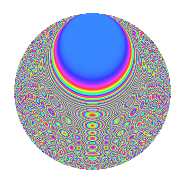# Properties

 Label 1575.2.bxLevel 1575 Weight 2 Character orbit bx Rep. character $$\chi_{1575}(64,\cdot)$$ Character field $$\Q(\zeta_{10})$$ Dimension 296 Sturm bound 480

# Related objects

## Defining parameters

 Level: $$N$$ = $$1575 = 3^{2} \cdot 5^{2} \cdot 7$$ Weight: $$k$$ = $$2$$ Character orbit: $$[\chi]$$ = 1575.bx (of order $$10$$ and degree $$4$$) Character conductor: $$\operatorname{cond}(\chi)$$ = $$25$$ Character field: $$\Q(\zeta_{10})$$ Sturm bound: $$480$$

## Dimensions

The following table gives the dimensions of various subspaces of $$M_{2}(1575, [\chi])$$.

Total New Old
Modular forms 992 296 696
Cusp forms 928 296 632
Eisenstein series 64 0 64

## Trace form

 $$296q + 72q^{4} + 10q^{5} + O(q^{10})$$ $$296q + 72q^{4} + 10q^{5} + 8q^{10} - 8q^{11} + 4q^{14} - 44q^{16} - 12q^{19} - 70q^{22} + 10q^{23} + 40q^{25} + 12q^{26} + 22q^{29} + 12q^{31} - 12q^{34} + 2q^{35} - 10q^{37} + 70q^{38} + 126q^{40} + 4q^{41} + 62q^{44} + 20q^{46} + 90q^{47} - 296q^{49} - 94q^{50} - 20q^{53} + 6q^{55} - 12q^{56} + 130q^{58} - 18q^{59} + 20q^{61} + 50q^{62} + 36q^{64} - 98q^{65} - 70q^{67} - 8q^{70} - 24q^{71} - 40q^{73} - 44q^{74} - 12q^{76} - 20q^{77} - 48q^{79} - 80q^{80} - 30q^{83} - 52q^{85} - 100q^{86} - 140q^{88} - 2q^{89} + 8q^{91} + 120q^{92} - 54q^{95} - 30q^{97} + O(q^{100})$$

## Decomposition of $$S_{2}^{\mathrm{new}}(1575, [\chi])$$ into newform subspaces

The newforms in this space have not yet been added to the LMFDB.

## Decomposition of $$S_{2}^{\mathrm{old}}(1575, [\chi])$$ into lower level spaces

$$S_{2}^{\mathrm{old}}(1575, [\chi]) \cong$$ $$S_{2}^{\mathrm{new}}(25, [\chi])$$$$^{\oplus 6}$$$$\oplus$$$$S_{2}^{\mathrm{new}}(75, [\chi])$$$$^{\oplus 4}$$$$\oplus$$$$S_{2}^{\mathrm{new}}(175, [\chi])$$$$^{\oplus 3}$$$$\oplus$$$$S_{2}^{\mathrm{new}}(225, [\chi])$$$$^{\oplus 2}$$$$\oplus$$$$S_{2}^{\mathrm{new}}(525, [\chi])$$$$^{\oplus 2}$$

## Hecke Characteristic Polynomials

There are no characteristic polynomials of Hecke operators in the database